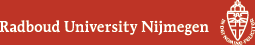## Geometry Seminar - Abstracts

### Talk

Monday 12 March 2018, 16:00-17:00 in HG00.058
Jérémie Joudioux (RU)
Introduction to the stability problem in mathematical relativity

### Abstract

The stability problem in general relativity is a very active field of research in the domain of mathematical relativity. It consists of the study of the mechanism of dispersion of a perturbation near a given solution to the Einstein equations. This mechanism is known in physics under the name of gravitational waves. If the full nonlinear stability of Minkowski spacetime, solution of the vacuum Einstein equations, has been proven at the begin of the 90s, the corresponding question for rotating black holes, the Kerr black holes, is today still an open question. Furthermore, in the presence of matter, the stability of flat spacetime remains an open problem for many types of matter models. The purpose of this talk is to introduce the stability problem in mathematical relativity as a PDE problem. The Cauchy problem for the Einstein equations is presented; then the question of the stability of Minkowski spacetime is addressed, and the basic ideas behind are presented. Finally, I will give a glimpse at the open problems, such as Kerr stability, or stability in the presence of matter.

(Back to geometry seminar schedule)